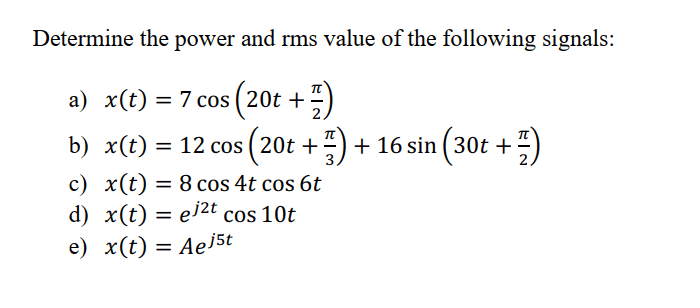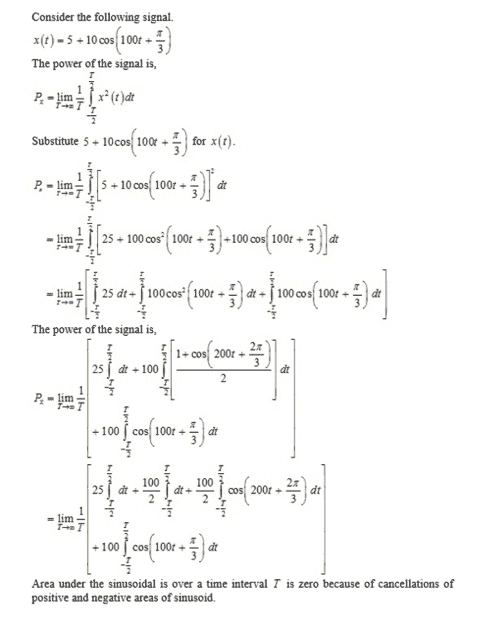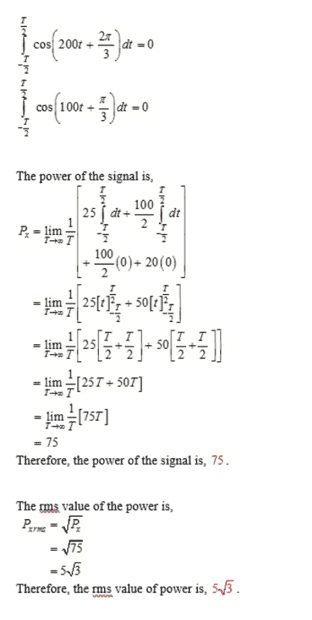# Power and RMS Value of a Signal

JamesBennettBeta
Homework Statement:
How to determine the power and RMS value of the given signals.
Relevant Equations:
$$P_{x} \ =\ \lim _{T\ \\rightarrow\infty } \ \frac{1}{T}\int _{-T/2}^{+T/2\ } x^{2} \ ( t) \ dt,$$

$$P_{x} \ =\ \frac{A^{2}}{2},$$
I need to calculate the power and RMS value of some equations. The problem is, I found two methods to do that and don't know which is the right method.

I have few equations to find the power and RMS value, but here is one equation.

$$x( t) \ =\ 7\cos\left( 20t+\frac{\pi }{2}\right),$$

Method #1

To find the power value,
$$P_{x} \ =\ \lim _{T\ \rightarrow\infty } \ \frac{1}{T}\int _{-T/2}^{+T/2\ } x^{2} \ ( t) \ dt ,$$

To find the RMS value,
$$P_{rms} \ =\ \sqrt{P_{x}},$$

This seems the right method for me, but later I found a YouTube video with a completely different approach.

Methods #2

To find the power value,

$$P_{x} \ =\ \frac{A^{2}}{2},$$

A is the amplitude of the equation.

To find the RMS value,

$$P_{rms} \ =\ \sqrt{P_{x}},$$

Homework Helper
Gold Member
2022 Award
Hi @JamesBennettBeta. Some of what you have written appears wrong/confusing. For example ##P_{rms} \ =\ \sqrt{P_{x}}## cannot be correct if “P” represents power, because the 2 sides of the equation would have different dimensions.
Maybe you are actually being asked to find the mean square value, and the rms value, of some function x(t). But that’s just a bit of a wild guess.

Can you post the complete, original question, word-for-word?

Last edited:
•JamesBennettBeta
JamesBennettBetaThanks for your reply, the question is this. A problem raised since I have found two ways to solve this. Anyway, I don't know either one is correct or not.

Method #1Method #2
Method two was explained in a video from an Indian Dr. I tried to solve the above problem using his way,

$$\begin{array}{l} 5+10 cos \left( 100t+\frac{\pi }{3}\right) Using\ the\ form\ A\ cos( \omega _{0} t\ +\ \theta )\\ Power\ of\ the\ signal,\\ \\ Px\ =\ \frac{A^{2}}{2} =\frac{10^{2}}{4} \ =\ 50w\\ \\ RMS\ value\ of\ the\ signal\\ \\ P_{RMS} \ =\ \sqrt{50} \ =\ 5\sqrt{2} \end{array}$$

The answers are completely different. I want to know what is the right method.

Homework Helper
Gold Member
2022 Award
A problem raised since I have found two ways to solve this. Anyway, I don't know either one is correct or not.
.
The answers are completely different. I want to know what is the right method.
A couple of general points first:

1. The rms value of a signal is not a power (it is the square-root of a power); it has the same dimensions as the signal itself. So do not use ##P_{rms}## as the symbol for the rms value of a signal; use (for example) ##x_{rms}##. So you could write ##x_{rms} = √P_x##.

(I will also note here that we are not using the term ‘power’ in the conventional sense of energy per unit time.)

2. The average value of sin(ωt+φ) or cos(ωt+φ) over a full cycle is zero. The average value of sin²(ωt+φ) or cos²(ωt+φ) over the same cycle is ½. These facts can save you a lot of work if correctly applied. (By applying them early, you can often save a lot of algebra.)
___________________________________

Your worked example is for x(t) = 5 + 10cos(100t + π/3). But this is not one of the questions you list (a – e). So I’m a bit confused a little by that.

Without checking your algebra detail, one point I note is that you appear to have incorrectly applied Method 2 when working out the power for x(t) = 5 + 10cos(100t + π/3).

I think that is the problem.

For a signal of the form:
x(t) = Acos(ωt + φ)
the average power is the average value of x² which is A²/2 (see point 2, above).

But for x(t) = 5 + 10cos(100t + π/3) you cannot take the average power to be A²/2 because of the constant term (5). For example the average power for
x(t) = 5 + 0.0001cos(100t + π/3) is a little over 5² = 25
(If that’s not clear, imagine a constant signal of x=5 units. The power is constant (25).)

And note you can't simply add the 25 to A²/2.

So method 2 will only work for some signals (but will save you a lot of time when correctly used). Method 1 will always work, but will require more work.

Sorry if that was a bit long-winded. Hope that it makes some sense!

•